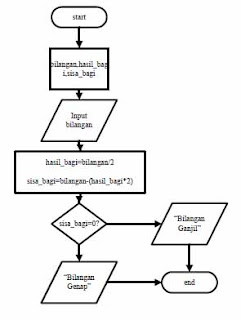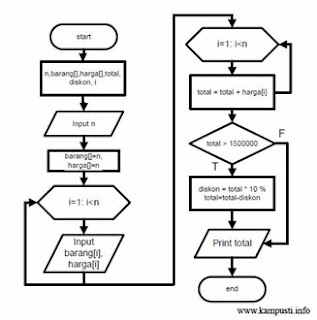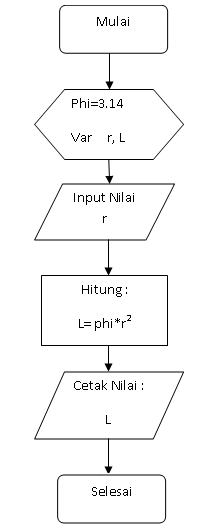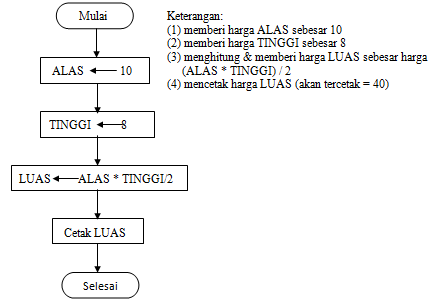Breaking News
Home / Computer / 7 Examples of Basic Programming Algorithms for Exercises# 7 Examples of Basic Programming Algorithms for Exercises

In the world of programming, mastering algorithms is important. Because the algorithm is the foundation to resolve a problem. Then, what is understanding of programming algorithm ?

Programming algorithm is a sequence of certain logical steps to solve a problem. This is emphasized on a logical step sequence, which means the algorithm must follow a certain sequence, and the steps should not be jumped. Another sense of the algorithm is the sequence of logical steps in systematically arranged problem solving.

The origin of the word algorithm itself comes from the name Abu Ja'far Mohammed Ibn Musa al-Khowarizmi, a Persian scientist who wrote a book called "Al Jabr W ' Al-Muqabala "(Rules of Restoration and Reduction) published in 825 M.

In the algorithm, the flow of thought in solving a problem is written in writing. The first thing to emphasize is the flow of thoughts, so one's algorithm can also be different from other people's algorithms. While the second emphasis is written, which means the groove can be a sentence, image, or a specific table.

The algorithm itself has some important features to be used to solve the problem, including:

• Algorithm must stop after doing a certain number of steps  Each step must be defined precisely and unambiguously
• The algorithm has an input of zero or more.
• The algorithm has an output of zero or more.
• The algorithm must be effective. That is, each written step should be simple so it can be done in a short time and makes sense.

In the computer field, algorithm function is indispensable for solving various programming problems, especially in numerical computation. Without a well-designed algorithm, the programming process would be wrong, corrupted, slow and inefficient. Implementing the steps in the algorithm is a computer system. In order for humans and computers to communicate, humans provide commands to the computer in the form of a collection of instructions that are collected in the program. In solving the problem, the computer needs to formulate several steps to solve the problem in a set of instructions. This collection of instructions understood by the computer is called the program.

To translate human language into computer language, a tool is needed to bridge communication between the two. The tool used is a programming language. Each programming language has its own level, depending on how the language can be applied directly by humans as users. Programming language levels can be categorized into 3 types, namely:

• High level Language (HLL). For example: Pascal, C, Java, PHP, ASP
• Medium Level Language (MLL). For example: Assembly
• Low level Language (LLL). For example: Machine Code

From various programming languages, how to give instructions are different. However, they all aim to produce the same output. Programs written in the programming language will be converted into machine language using the translator. Here is a method of translating programming languages ​​into machine language in programming:

• Interpreter, ie translating lines per instruction line. Basic language uses this method.
• Compiler, ie translates after all the written instructions. Pascal, and C are some examples of programming languages ​​that use this method.

In studying programming, you must understand the difference between learning programming and learning programming languages. Learning programming means you learn about the problem-solving method, then pour it in a certain notation that is easy to read and understand. While learning programming language means you learn to use a language, grammar rules, instruction used, and the procedure of operating the compiler, to create programs written into the language.

The writing of the algorithm must consist of the following three parts:  Title of the algorithm; Section consisting of algorithm names and explanations (specifications) about the algorithm. The name should be brief and describe what the algorithm does.

• Declaration; Section to define all the names used in the program. The name may be a name of a constant, a variable, a type, a procedure and a function.
• Description; This section contains a description of the problem-solving steps written using the notation that will be described next.
• The writing of the algorithm itself is independent of the specification of the programming language and the capabilities of the computer that executes it. The algorithm notation is not a programming language notation, but the algorithm can be translated into various programming languages. Then what kind of example of basic programming algorithm you must master? Here are some examples:

1. Narrative Algorithm

Issue: Given data in the form of student's name and value. If the student score is greater than or equal to 60 then the student is declared graduated. If the value is less than 60, then the student is declared not pass.

The algorithm will be as follows:
read the name and value of student
if value> = 60 then
description = pass
but if
information = not pass.
write the name and description

2. Pseudo Code Algorithm

Example; Algorithm Kelulusan_mhs

Issue: Given data in the form of student's name and value. If the student score is greater than or equal to 60 then the student is declared graduated. If the value is less than 60, then the student is declared not pass.

Declaration of the data type will be as follows:
Name = string
Value = integer
Description = string

The algorithm will be like the following
if the value> = 60 then
the information = 'pass'
else
description = 'not pass'
write (name, description)

3. Flowchart Algorithm

The following are some examples of flowchart algorithms. The function of flowchart on programming is to facilitate programmers when designing a computer program. Here are the examples:

• Determining an odd number or even number

There are numbers named "integers" that are 0, 1, -1, 2, and so on and native numbers 1, 2, 3, 4, 5, etc. Both types of numbers are often used in calculations. The algorithm in this section will determine whether the number is odd or even> The following is the flowchart of the algorithm:• Calculating the price to pay after getting a discount

Sample flowchart times this is the flowchart of the algorithm to determine the cost to be paid by the buyer after getting 10% discount. The requirement to get the discount is the amount of the total purchase of Rp.1.500.000, -. The following is the flowchart algorithm:The number of items has a dynamic nature in accordance with the input of the user. In essence, if the total purchase is less than 1500000, the buyer will not get a discount.

• Flowchart calculate the area of ​​the circle

The flowchart algorithm below is a flowchart example to calculate the area of ​​a circle with the formula L = πr2:

Algorithm:  Calculate the value of phi and r

• Calculate L = phi x r2
• Print Result L
• Program Completed
• Flowchart:• Flowchart calculates area of ​​triangle

Here  an example of a flowchart program to calculate the area of ​​a triangle, where it is known that the value of ALAS = 10 and the value of HIGH = 8. In this flowchart, to get the initial value of "ALAS" and "HIGH" using process box , since the values ​​of "ALAS" and "HIGH" have been predetermined.All of our articles this time around the example of basic programming algorithms. Hopefully our article this time can be your material to learn basic programming.